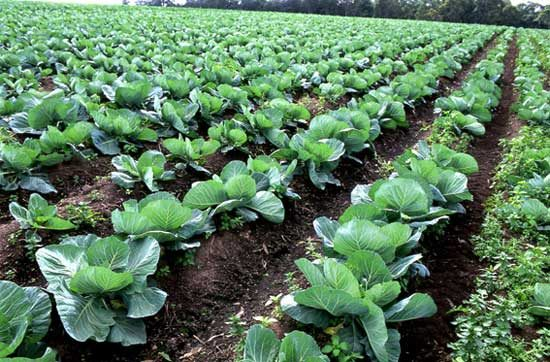# AGRIC6: Agricultural Production Economics: Basic Concepts

##### This unit looks at the basic concepts of agricultural production economics1. Production: The process through which some goods and services called inputs are transformed into other goods called products or
2. Production function: A systematic and mathematical expression of the relationship among various quantities of inputs or input services used in the production of a commodity and the corresponding quantities of output is called a production
3. Continuous production function: This function arises for those inputs which can be divided into smaller doses. Continuous variables can be known from measurement, for example, seeds and fertilizers,
4. Discontinuous or discrete production function: This function arises for those inputs or work units which cannot be divided into smaller units and hence are used in whole numbers. For example, number of ploughings, weedings and harvestings,
5. Short run production period: The planning period during which one or more of the resources are fixed while others are variable resources. The output can be varied only by intensive use of fixed resources. It is written as

Y=f (X1, X2 / X3…..Xn) where Y is output, X1, X2 are variable inputs and X3…..Xn are fixed inputs.

1. Long run production period: The planning period during which all the resources can be It is written as

Y=f (X1, X2 ,…..Xn)

1. Technical coefficient: The amount of input per unit of output is called technical coefficient.
2. Resources: Anything that aids in production is called a resource. The resources physically enter the production
3. Resource services: The work done by a person, machine or livestock is called a resource service. Resources do not enter the production process
1. Fixed resources: The resources that remain unchanged irrespective of the level of production are called fixed resources. For example, land , building, machinery. These resources exist only in short run. The costs associated with these resources are called fixed
2. Variable resources: The resources that vary with the level of production are called variable resources. These resources exist both in short run and long For example, seeds, fertilizers, chemicals, etc. The costs associated with these resources are called variable costs.
3. Flow resources: The resources that cannot be stored and should be used as and when these are available. For example, services of a labourer on a particular
4. Stock resources: The resources that can be stored for use later on. For example, seeds. Defining an input as a flow or stock depends on the length of time under consideration. For example, tractor with 10 years life is a stock resources if we take the services of tractor for its entire useful life of 10 But it also provides its service every day, therefore it is a flow resources.
5. Production period: It is the time period required for the transformation of resources or inputs into products.
6. Farm entrepreneur: Farm entrepreneur is the person who organizes and operates the farm business and bears the responsibility of the outcome of the
7. Farm business manager: Person appointed by the entrepreneur to manage and supervise the farm business and is paid for the services rendered. He/she carries out the instructions of the
8. Productivity: Output per unit of inputs is called the
9. Technical efficiency: It is the ratio of the physical output to inputs used. It implies the using of resources as effectively as possible.
10. Economic efficiency: It is the expression of technical efficiency in monetary terms through the prices. In other words, the ratio of value of output to value of inputs is termed as economic efficiency.
1. Allocative efficiency: It occurs when no possible reorganization of resources/production can make any combination higher yielding without making other combination less yielding. It refers to resource use
2. Optimality: It is an ideal condition or situation in which costs are minimum and/or profits
3. Cost of cultivation: The expenditure incurred on all inputs and input services in raising a crop on a unit area is called cost of cultivation. It is expressed as rupees per hectare or rupees per
4. Cost of production: The expenditure incurred in producing a unit quantity of output is known as cost of production, for example, Rs./kg of /quintal.
5. Independent variable: Variable whose value does not depend on other variables and which influences the dependent variable, is termed as independent variable, for example, land, labour and
6. Dependent variable: Variable whose value depends on other variables is termed as dependent variable, for example, crop
7. Slope of a line: It represents the rate of change in one variable that occurs when another variable changes. Slope varies at different points on a curve but remains same on all points on a given line. It is the rate of change in the variable on vertical axis per unit change in the variable on horizontal axis and is expressed as a number.
8. Total physical product: Total amount of output obtained by using different units of inputs measured in physical units, for example, kg, tonnes,
9. Average physical product (APP): Output per unit of input on an average is termed as APP and is given by Y/X.

Marginal physical product: Addition to total output obtained by using the marginal unit of input and is measured as ΔY/ΔX.

SEE ALL
•YOU